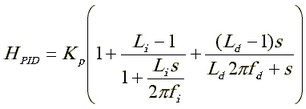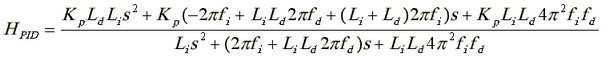﻿ 20-sim webhelp > Toolboxes > Control Toolbox > Filter Editor > Controllers > PID1-Controller

# PID1-Controller

The PID-1 Controller consists of an integrator and differentiator with proportional gain. It resembles a PID Controller, but has different input parameters.

 • Gain Kp: The controller gain Kp determines the frequency gain of the controller in the pass band (where integration and differentiation are of no interest).
 • Length Li: The length Li determines the frequency at which integration starts, that is fi / Li in Hz. A decrease of this frequency corresponds to a more pure integration behavior.
 • Length Ld: The length Ld determines the frequency at which differentiation stops, that is fd * Ld in Hz. An increase of this frequency corresponds to a more pure differentiation behavior, reducing the tameness of the controller.
 • Frequency fi: The integration behavior of the controller stops at frequency fi in Hz.
 • Frequency fd: The differentiation behavior of the controller starts at frequency fd in Hz.

## Transfer function of the controller:that equals